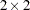Introduction to Categorical Data Analysis Procedures
 Relaxation of Sampling Assumptions

As indicated previously, the modeling procedures assume that the data are from a stratified simple random sample, so they use the product multinomial distribution. If the data are not from such a sample, then in many cases it is still possible to use a modeling procedure by arguing that each row of the contingency table does represent a simple random sample from some hypothetical population. The extent to which the inferences are generalizable depends on the extent to which the hypothetical population is perceived to resemble the target population.

Similarly, the Cochran-Mantel-Haenszel statistics use the multiple hypergeometric distribution, which requires fixed row and column marginal totals in each contingency table. If the sampling process does not yield a table with fixed margins, then it is usually possible to fix the margins through conditioning arguments similar to the ones used by Fisher when he developed the Exact Test fortables. In other words, if you want fixed marginal totals, you can generally make your analysis conditional on those observed totals.

For more information on sampling models for categorical data, see Bishop, Fienberg, and Holland (1975, Chapter 13) and Agresti (2002, Chapter 1.2).Previous Page | Next Page | Top of Page